Refer to our Texas Go Math Grade 5 Answer Key Pdf to score good marks in the exams. Test yourself by practicing the problems from Texas Go Math Grade 5 Lesson 1.7 Answer Key Add and Subtract Decimals Through Thousandths.

Unlock the Problem

At the 2010 Winter Olympics, Armin Zoeggeler r won the bronze medal in the men’s luge event, r In his fastest run, he completed the first interval in 21.261 seconds. It took him another 27.198 b seconds to reach the finish line. What was Zoeggeler’s finish time?

• Underline the sentence that tells you what you are trying to find.
• Circle the numbers you need to use.

Add decimals through thousandths. 21.261 + 27.198
THINK

• Line up the numbers in each place.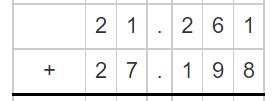• Then, add the hundredths, tenths, ones, and tens. Regroup as needed.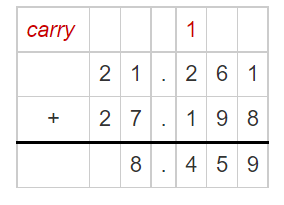• Place the decimal point in the sum.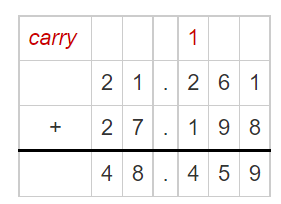So,
Zoeggeler’s finish time was 48.459 seconds.

Math Talk
Mathematical Processes

Why would you use paper and pencil instead of mental math to solve this problem?
We can use mental math to solve simple equations. To do this, we put the equation into words and then form a question with those words. We then answer the question in our heads. We can also make use of inverse operations to reword questions if the answer isn’t obvious to us.
But,
In the above situation,
It is not possible because the given numbers are decimal numbers with three digits after the decimal point

Try This!

Use subtraction to check your work. Subtract one of the addends from the sum.

The given numbers from the above problem are: 21.261 and 27.198
Now,
To find out the answer to the above problem is correct or not,
We have to subtract the answer from one of the given numbers to get the second number,
If we didn’t get the second number, then the answer is not correct
So,
48.459 – 27.198 = 21.261
Hence, from the above,
We can conclude that
The answer to the above problem is correct

Example
Zoeggeler finished the first half of the run in 31.116 seconds. The second half took only 17.343 seconds. How many seconds faster was the second half of the run?
Estimate. 31 – 17 = ___________

STEP 1
Line up the place values. Subtract the thousandths.STEP 2
Subtract the hundredths. Subtract the tenths. Regroup as needed.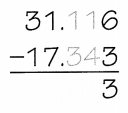STEP 3
Subtract the ones and tens. Place the decimal point in the difference.So,
Zoeggeler was 13.773 seconds faster in the second half of the run.

To check subtraction using the inverse operation, add the number you subtracted to the difference. The sum should equal the number you subtracted from.

Math talk
Mathematical Processes

Explain how you know without adding that the sum of 2.475 and 6.43 will result in a 5 in the thousandths place.
The given numbers are: 2.475 and 6.43
Now,
When we observe the given numbers,
2.475 has the thousandths place value but 6.43 does not have the thousandths place value
So,
The representation of 6.43 with the thousandths place will be: 6.430
Now,
When we add the thousandths place of both the numbers, i.e., 5 + 0,
We will get the result as 5
Hence, from the above,
We can conclude that
To make the numbers have the same place values when there are unequal place values, add zeros to the right side of the number that has the number of place values less than the other number

Try This! Subtract. Then check your work.

Question 1.The given numbers are: 42.784 and 6.980
So,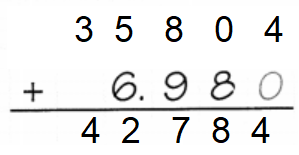Share and Show

Find the sum or difference.

Question 1.The given numbers are: 1.845 and 0.357
So,Hence, from the above,
We can conclude that
The result for the given expression is: 1.488

Question 2.
3.46 + 6.834
The given numbers are: 3.46 and 6.834
So,Hence, from the above,
We can conclude that
The result for the given expression is: 10.294

Question 3.
13 – 0.943
The given numbers are: 13 and 0.943
So,Hence, from the above,
We can conclude that
The result for the given expression is: 12.057

Question 4.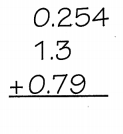The given numbers are: 0.254, 1.3, and 0.79
So,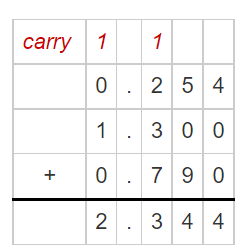Hence, from the above,
We can conclude that
The result for the given expression is: 2.344

Problem Solving

Find the sum or difference.

Question 5.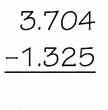The given numbers are: 3.704 and 1.325
So,Hence, from the above,
We can conclude that
The result for the given expression is: 2.379

Question 6.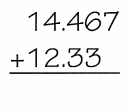The given numbers are: 14.467 and 12.33
So,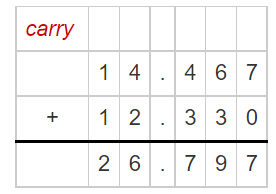Hence, from the above,
We can conclude that
The result for the given expression is: 26.797

Question 7.The given numbers are: 23.002 and 1.74
So,Hence, from the above,
We can conclude that
The result for the given expression is: 21.262

Question 8.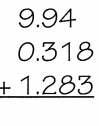The given numbers are: 9.94, 0.318, and 1.283
So,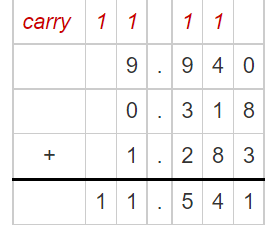Hence, from the above,
We can conclude that
The result for the given expression is: 11.541

Practice: Copy and Solve Find the sum or difference.

Question 9.
21.54 + 4.758
The given numbers are: 21.54 and 4.758
So,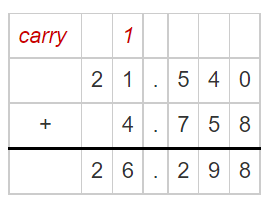Hence, from the above,
We can conclude that
The result for the given expression is: 26.298

Question 10.
6.328 – 3.62
The given numbers are: 6.328 and 3.62
So,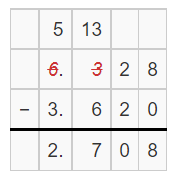Hence, from the above,
We can conclude that
The result for the given expression is: 2.708

Question 11.
15.87 – 3.274
The given numbers are: 15.87 and 3.274
So,Hence, from the above,
We can conclude that
The result for the given expression is: 12.596

Question 12.
2.45 + 3.247 + 1.8
The given numbers are: 2.45, 3.247, and 1.8
So,Hence, from the above,
We can conclude that
The result for the given expression is: 7.497

H.O.T. Algebra Find the unknown numbers in the pattern. Then write a description for the pattern.

Question 13.
2.1, 3.3, 4.5, 5.7, ______ , 8.1, ________
Description: _____________
The given pattern is:
2.1, 3.3, 4.5, 5.7, ______ , 8.1, ________
Now,
When we observe the above pattern,
We can say that
2.1 + 1.2 = 3.3
3.3. + 1.2 = 4.5
Hence, from the above,
We can conclude that
The completed pattern is:
2.1, 3.3, 4.5, 5.7, 6.9 , 8.1, 9.3
We have to add 1.2 to the previous number to complete the given pattern

Question 14.
4.05, 4.00, 3.95, ________, 3.85, ________
Description: ______________
The given pattern is:
4.05, 4.00, 3.95, ________, 3.85, ________
Now,
When we observe the above pattern,
We can say that
4.05 – 0.05 = 4.00
4.00 – 0.05 = 3.95
Hence, from the above,
We can conclude that
The completed pattern is:
4.05, 4.00, 3.95, 3.90, 3.85, 3.80
We have to subtract 0.05 from the previous number to complete the given pattern

Problem Solving

Use the table to solve 15-16.Question 15.
Evaluate Apolo Ohno won the men’s 500-meter speed skating final at the 2006 Winter Olympics. His time for the race was 4 1.935 seconds. Francois-Louis Tremblay came in second, finishing 0.067 second behiñd Ohno. What was Tremblay’s time?
It is given that
Apolo Ohno won the men’s 500-meter speed skating final at the 2006 Winter Olympics. His time for the race was 4 1.935 seconds. Francois-Louis Tremblay came in second, finishing 0.067 second behiñd Ohno
Now,
The given table is:So,
The time is taken by Tremblay to complete the final = 41.935 – 0.067
Now,
By using the Long Subtraction,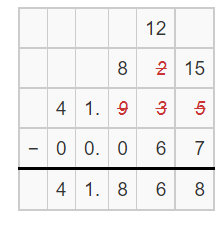Hence, from the above,
We can conclude that
The time took by Tremblay to complete the final is: 41.868 seconds

Question 16.
Apply Jon Eley came in fifth in the men’s speed skating final. How many seconds after Apolo Ohno did Eley finish?
It is given that
Jon Eley came in fifth in the men’s speed skating final
Now,
The given table is:So,
The time took Eley to finish the final after Apolo Ohno = 42.497 – 41.935
Now,
By using the Long subtraction,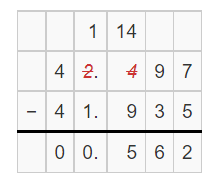Hence, from the above,
We can conclude that
The time took Eley to finish the final 0.0562 seconds after Apolo Ohno

Question 17.
H.O.T. Multi-Step The sum of two numbers is 4.004. One number has a 4 in the tenths place and a 3 in the thousandths place. The other number has a 1 in the one’s place and an 8 in the hundredths place. What are the two numbers?It is given that
The sum of two numbers is 4.004. One number has a 4 in the tenths place and a 3 in the thousandths place. The other number has a 1 in the one’s place and an 8 in the hundredths place.
Now,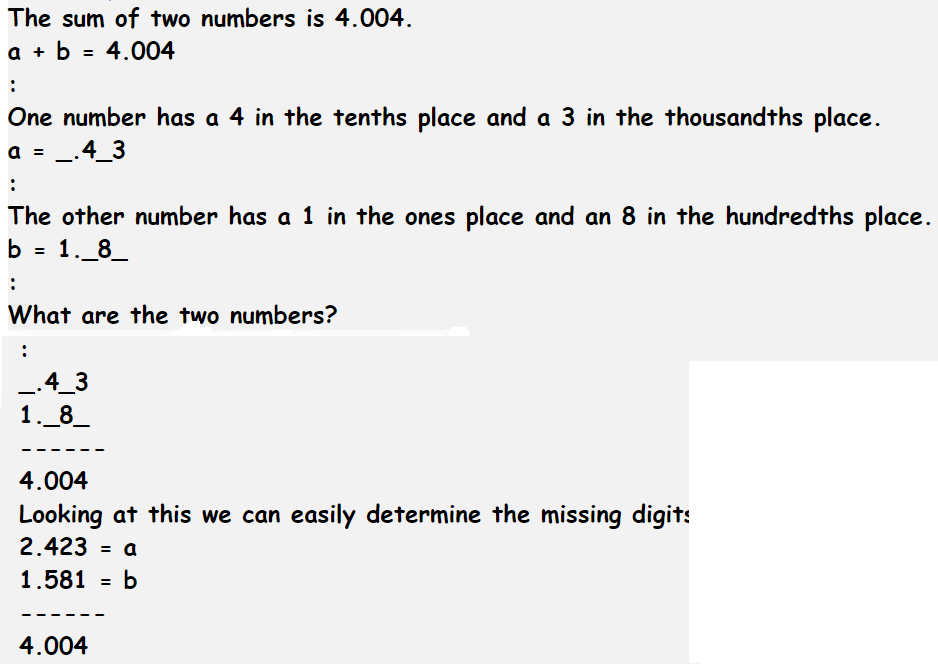Hence, from the above,
We can concldue that
The two numbers are: 2.423 and 1.581

Question 18.
Multi-Step Team A spent 61.242 seconds and 59.438 seconds running a relay race. Team B spent 60.561 seconds and 60.289 seconds running the relay race. Which statement is true?
A. Team A won by 0.17 seconds.
B. Team B won by 0.17 seconds.
C. Team A won by 1.17 seconds.
D. Team B won by 1.17 seconds.
It is given that
Team A spent 61.242 seconds and 59.438 seconds running a relay race. Team B spent 60.561 seconds and 60.289 seconds running the relay race
Now,
According to the given information,
The  time taken by Team A running the relay race = 61.242 – 59.438
= 1.804 seconds
The  time taken by Team B running the relay race = 60.561 – 60.289
= 0.272 seconds
So,
The difference between the times to finish the relay race = 1.804 – 0.272
= 1.532 seconds
Hence, from the above,
We can conclude that
The statements that are true is:Question 19.
Manuel is walking from his home to his school. He has walked 0.142 miles so far and has 0.088 miles left to walk. How far is Manuel’s home from school?
A. 0.054 mile
B. 0.12 mile
C. 0.23 mile
D. 1.022 miles
It is given that
Manuel is walking from his home to his school. He has walked 0.142 miles so far and has 0.088 miles left to walk
So,
According to the given information,
The distance of Manuel’s home from school = 0.142 + 0.088
= 0.230 miles
Hence, from the above,
We can conclude that
The distance of Manuel’s home from school is:Question 20.
Multi-Step Music & Movie Mart is selling used CDs for $6.99 each and used DVDs for$8.49 each. You have $30 to spend. Which of these combinations could you buy? A. TWO CDS and two DVDs B. Three CDs and one DVD C. Three CDs and three DVDs D. One CD and three DVDs Answer: It is given that Music & Movie Mart is selling used CDs for$6.99 each and used DVDs for $8.49 each. You have$30 to spend.
Now,
By using the options,
A.
2 ($6.99) + 2 ($8.49) = $30.96 B. 3 ($6.99) + $8.49 =$29.46
C.
3 ($6.99) + 3 ($8.49) = $46.44 D.$6.99 + 3 ($8.49) =$32.46
Hence, from the above,
We can conclude that
The combinations that you could buy is: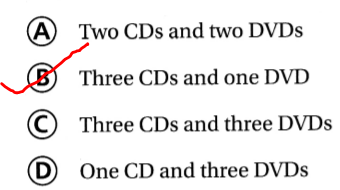Texas Test Prep

Question 21.
Toni has a ribbon that is 2.75 meters long. She cuts off 0.345 meters. How much of the ribbon does Toni have left?
A. 3.095 meters
B. 2.715 meters
C. 2.785 meters
D. 2.405 meters
It is given that
Toni has a ribbon that is 2.75 meters long. She cuts off 0.345 meters
So,
The amount of ribbon does Toni have left = 2.75 – 0.345
= 2.405 meters
Hence, from the above,
We can conclude that
The amount of ribbon does Toni have left is:### Texas Go Math Grade 5 Lesson 1.7 Homework and Practice Answer Key

Find the sum or difference.

Question 1.The given numbers are: 13.87 and 6.06
So,Hence, from the above,
We can conclude that
The result for the given addition expression is: 19.93

Question 2.The given numbers are: 26.25 and 5.73
So,Hence, from the above,
We can conclude that
The result for the given subtraction expression is: 20.52

Question 3.The given numbers are: 2.50 and 0.926
So,Hence, from the above,
We can conclude that
The result for the given addition expression is: 3.426

Question 4.The given numbers are: 43.66 and 9.08
So,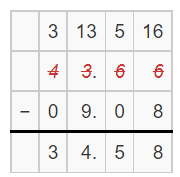Hence, from the above,
We can conclude that
The result for the given subtraction expression is: 34.58

Question 5.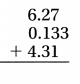The given numbers are: 6.27, 0.133, and 4.31
So,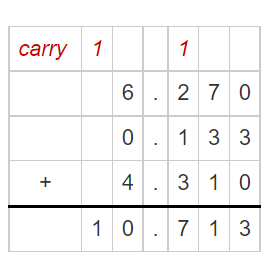Hence, from the above,
We can conclude that
The result for the given addition expression is: 10.713

Question 6.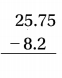The given numbers are: 25.75 and 8.2
So,Hence, from the above,
We can conclude that
The result for the given subtraction expression is: 17.55

Find the sum or difference.

Question 7.
6.389 + 17.39
The given numbers are: 6.389 and 17.39
So,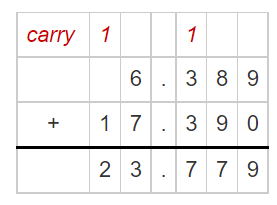Hence, from the above,
We can conclude that
The result for the given addition expression is: 23.779

Question 8.
8.747 – 4.8
The given numbers are: 8.747 and 4.8
So,Hence, from the above,
We can conclude that
The result for the given subtraction expression is: 3.947

Question 9.
2.09 + 12.639
The given numbers are: 2.09 and 12.639
So,Hence, from the above,
We can conclude that
The result for the given addition expression is: 14.729

Find the unknown numbers in the pattern. Then write a description for the pattern

Question 10.
1.0, 2.1, 3.2, 4.3. ________ 6.5, 7.6
Description: _____________
The given pattern is:
1.0, 2.1, 3.2, 4.3. ________ 6.5, 7.6
Now,
From the given pattern,
We can observe that
1.0 + 1.1 = 2.1
2.1 + 1.1 = 3.2
Hence, from the above,
We can conclude that
The completed pattern is:
1.0, 2.1, 3.2, 4.3. 5.4, 6.5, 7.6
The description of the given pattern is:
Add 1.1 to the first number and continue the same pattern

Question 11.
5.03,5.00, 4.97, 4.94 ________ 4.88
Description: _____________
The given pattern is:
5.03,5.00, 4.97, 4.94 ________ 4.88
Now,
From the above pattern,
We can observe that
5.03 – 0.03 = 5.00
5.00 – 0.03 = 4.97
Hence, from the above,
We can conclude that
The completed pattern is:
5.03,5.00, 4.97, 4.94, 4.91, 4.88
The description of the given pattern is:
Subtract 0.03 from the first number and continue the same pattern

Problem Solving

Question 12.
Abby finished a biking race in 21.39 minutes. Juanita took 19.59 minutes to finish. How much longer did Abby take to finish the race than Juanita?
It is given that
Abby finished a biking race in 21.39 minutes. Juanita took 19.59 minutes to finish.
So,
The difference between the time took by Abby and Junaita = 21.39 – 19.59
Now,
By using the Long Subtraction,Hence, from the above,
We can conclude that
Abby took 1.80 seconds more than Junaita to finish the race

Question 13.
The sum of the two numbers is 5.036. One number has a 4 in the tenths place and a 7 in the thousandths place. The other number has a 1 in the one’s place and a 2 in the hundredths place. What are the two numbers?
It is given that
The sum of the two numbers is 5.036. One number has a 4 in the tenths place and a 7 in the thousandths place. The other number has a 1 in the one’s place and a 2 in the hundredths place.
Now,Hence, from the above,
We can conclude that
The two numbers are: 1.629 and 3.407

Lesson Check

Question 14.
Dara spends $13.02 on sandals,$9.85 on shorts, and $15.20 on a hat. How much change does she receive back from$50?
A. $10.93 B.$11.93
C. $11.34 D.$12.67
It is given that
Dara spends $13.02 on sandals,$9.85 on shorts, and $15.20 on a hat. So, According to the given information, The amount of money Dara received back from$50 = $50 – ($13.02 + $9.85 +$15.20)
= $50 –$38.07
= $11.93 Hence, from the above, We can conclude that The amount of money Dara received back from$50 is:Question 15.
Ling’s relay team ran the first part of the race in 28.134 seconds, the second part in 17.922 seconds, and the third part in 34.023 seconds. By how much did the relay team beat the champions, who ran the race in 83.736 seconds?
A. 3.412 seconds
B. 3.657 seconds
C. 3.327 seconds
D. 4.073 seconds
It is given that
Ling’s relay team ran the first part of the race in 28.134 seconds, the second part in 17.922 seconds, and the third part in 34.023 seconds
So,
According to the given information,
The difference of time between Ling;s team and champions = 83.736 – (28.134 + 17.922 + 34.023)
= 3.657 seconds
Hence, from the above,
We can conclude that
The difference of time between the relay team and the Champions is: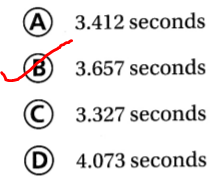Question 16.
Juan has a batting average of 0.334. Gary has a batting average of 0.284. What is the difference between the batting averages of the two baseball players?
A. 0.50
B. 0.05
C. 0.028
D. 0.042
It is given that
Juan has a batting average of 0.334. Gary has a batting average of 0.284
Now,
The difference between the batting averages of the two baseball players = 0.334 – 0.284
= 0.050 seconds
Hence, from the above,
We can conclude that
The difference between the batting averages of the two baseball players is:Question 17.
Jonas is jogging from the park to the school. He has jogged 0.424 miles so far. He has 0.384 miles left to jog. How far is the park located away from the school?
A. 0.730 mile
B. 0.808 mile
C. 1.032 miles
D. 0.40 mile
It is given that
Jonas is jogging from the park to the school. He has jogged 0.424 miles so far. He has 0.384 miles left to jog.
So,
According to the given information,
The distance from the park to the school = 0.424 + 0.384
= 0.808 miles
Hence, from the above,
We can conclude that
The distance from the park to the school is: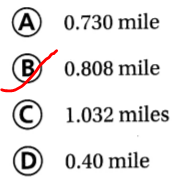Question 18.
Multi-Step Jimmie adds 3.24, 4.294, and 2.073. How much more than 5.31 is the sum?
A. 4.297
B. 9.607
C. 4.607
D. 4.477
It is given that
Jimmie adds 3.24, 4.294, and 2.073
So,
According to the given information,
The difference between 5.31 and the numbers that will be added by Jimmie = (3.24 + 4.294 + 2.073) – 5.31
= 4.297
Hence, from the above,
We can conclude that
The difference between 5.31 and the numbers that will be added by Jimmie is:Question 19.
Multi-Step A gallon of milk costs $3.32 and a container of orange juice costs$2.95. Max buys two gallons of milk and two containers of orange juice. How much does Max spend?
A. $123.69 B.$6.64
C. $12.54 D.$6.27
A gallon of milk costs $3.32 and a container of orange juice costs$2.95. Max buys two gallons of milk and two containers of orange juice
The total amount of money spent by Max = 2 ($3.32) + 2 ($2.95)
= $6.64 +$5.9